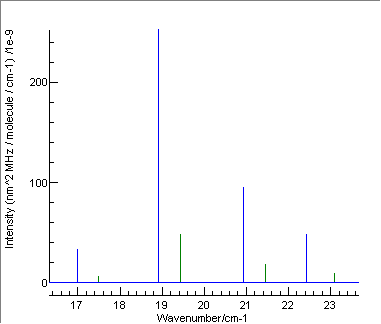Molecule Types Linear Molecules Samples

# O2 Microwave Spectrum

## Main Isotope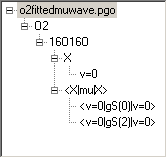This shows an example of a microwave spectrum using a magnetic moment. The values are taken from G.Yu. Golubiatnikov and A.F. Krupnov, J Molec. Spectrosc. 225, 222 (2004), but with γH = 0, which gives a better fit to the experimental data. It gives good, but not exact agreement with their calculated values. The values calculated by PGOPHER have been checked against the explicit formulae given in Rouille at al, J Molec. Spectrosc. 154, 372 (1992) so the discrepancy is probably due to a slight difference in the method of calculation. Data file: o2fittedmuwave.pgo. Note the following:
• Symmetric = True for the molecule
• AsymWt = 0 to reflect the fact that half the rotational levels are missing as 16O has spin 0.
• The definition of the origin of the energy scale is the subject of some confusion in the literature - see T. G. Slanger and P. C. Cosby, J. Phys. Chem., 92, 267 (1988) doi:10.1021/j100313a008. The definition used here is equivalent to that given in the paper, with the lowest level (J=0, N=1, e parity) at +0.2458cm-1.
• This lowest level (J=0, N=1, e parity) is labelled F1 by default in PGOPHER, though F3 is also used in the literature. This is because PGOPHER applies the F1 and F3 labels by energy order, rather than on J-N. This only makes a difference for J < S, which here implies J=0 only; there is no ambiguity for the higher levels. The choice of labels for the lowest level can be switched with the OmegaOrder setting; the default of Auto implies the state is Regular (as LambdaSS is positive) but setting it to Inverted switches the omitted F label, so the lowest level is labelled F3. The only effect this has on the other levels is to swap the  Ω=0 and 1 labels for e levels. (These are only shown if ShowOmega is set.). Note that for correct operation of OmegaOrder, LimitSearch=True is required, which is the default for new files. Note that earlier versions of this sample file did not have this set.

## 18O16O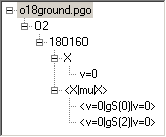This is the microwave spectrum of the 18O16O species. The constants were obtained by fitting to the observed microwave lines given in the JPL  Molecular Spectroscopy catalog (H. M. Pickett, R. L. Poynter, E. A. Cohen, M. L. Delitsky, J. C. Pearson, and H. S. P. Muller, "Submillimeter, Millimeter, and Microwave Spectral Line Catalog," J. Quant. Spectrosc. & Rad. Transfer 60, 883 (1998)) λH was fixed to the value for 16O2.
Data file: o18ground.pgo Note the following differences:
• Symmetric = False for the molecule (no u/g for this isotope) and AsymWt = 1 as nuclear spin statistics have no effect here

## 17O16O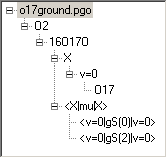This is the microwave spectrum of the 17O16O species. The constants were obtained by fitting to the data from G. Cazzoli et al., Nuovo Ciminto 62, 243 (1981) obtained from the JPL  Molecular Spectroscopy catalog. The centrifugal distortion constants were fixed to the value for 16O2.

Data file: o17ground.pgo Note the following differences:
• Symmetric = False for the molecule (no u/g for this isotope)
• nNuclei = 1, as 17O has spin 5/2 (and 3 hyperfine parameters are determined by the data).

## All isotopes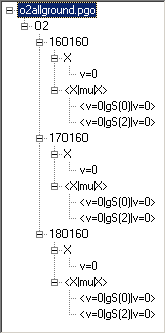This file combines all the above. Data file: o2allground.pgo Note the following differences:
• Abundance has been set as appropriate
• The hyperfine structure has been turned off in the 17O16O species to simplify the spectrum.
• Colours have been set on the spectrum so that individual spectra will show in different colours, as in the sample below (Green = 17O16O, Blue = 18O16O; the main isotope is in red but does not show in this picture).